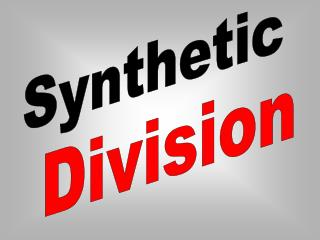DownloadDownload PresentationSynthetic

Synthetic

Télécharger la présentationSynthetic

- - - - - - - - - - - - - - - - - - - - - - - - - - - E N D - - - - - - - - - - - - - - - - - - - - - - - - - - -
Presentation Transcript

1. Synthetic Division

2. Let’s look at how to do this using the example: In order to use synthetic division these two things must happen:

3. There must be a coefficient for every possible power of the variable. The divisor must have a leading coefficient of 1.

4. Since the numerator does not contain all the powers of x, you must include a zero for Step #1: Write the terms of the polynomial so the degrees are in descending order.

5. Step #2: Write the constant r of the divisor x - r to the left and write down the coefficients. . Since the divisor = x - 3, r=3 5 0 -4 1 6

6. and place under the second coefficient then add. Step #4: Multiply the first coefficient by r, so 5 15 15

7. Step #5: Multiply the sum, 15, by r; and place this number under the next coefficient then add. 15 15 5 45 41

8. 45 15 41 15 5 Step #6: Repeat the same procedure as step #5. 123 372 124 378 Where did 123 and 372 come from?

9. Step #7: Write the quotient. The numbers along the bottom are coefficients of the power of x in descending order, starting with the power that is one less than that of the dividend.

10. The quotient is: Remember to place the remainder over the divisor.

11. Try these: